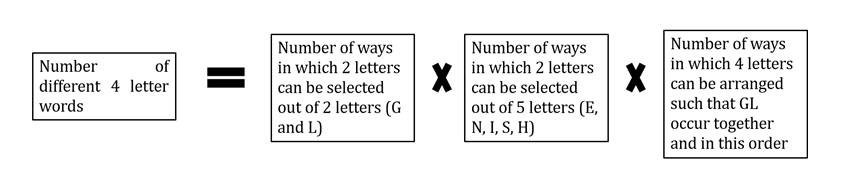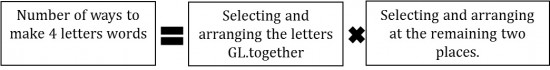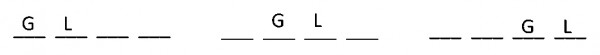GMAT Question of the Day - Daily to your Mailbox; hard ones only

 It is currently 23 Oct 2019, 06:41GMAT Club Daily Prep

Thank you for using the timer - this advanced tool can estimate your performance and suggest more practice questions. We have subscribed you to Daily Prep Questions via email.

Customized
for You

we will pick new questions that match your level based on your Timer History

Track

every week, we’ll send you an estimated GMAT score based on your performance

Practice
Pays

we will pick new questions that match your level based on your Timer History

Not interested in getting valuable practice questions and articles delivered to your email? No problem, unsubscribe here.How many 4-letter words can be formed using the alphabets of the word

 new topic post reply Question banks Downloads My Bookmarks Reviews Important topics
Author Message
TAGS:

Hide Tags

e-GMAT RepresentativeV
Joined: 04 Jan 2015
Posts: 3092
How many 4-letter words can be formed using the alphabets of the word  [#permalink]

Show Tags

2
800:00

Difficulty:55% (hard)

Question Stats:60% (01:37) correct40% (01:55) wrongbased on 164 sessions

HideShow timer Statistics

How many 4-letter words can be formed using the alphabets of the word ENGLISH, if it is given that the 4-letter word contains alphabets G and L paired together, with G coming before L and repetition of alphabets being not allowed?

A. $$60$$
B. $$120$$
C. $$180$$
D. $$200$$
E. $$240$$

Thanks,
Saquib
Quant Expert
e-GMAT

Register with us to get access to free content_________________

Originally posted by EgmatQuantExpert on 30 Jan 2017, 00:34.
Last edited by EgmatQuantExpert on 07 Aug 2018, 03:13, edited 1 time in total.
e-GMAT RepresentativeV
Joined: 04 Jan 2015
Posts: 3092
Re: How many 4-letter words can be formed using the alphabets of the word  [#permalink]

Show Tags

The official solution has been posted. Looking forward to a healthy discussion.._________________

Originally posted by EgmatQuantExpert on 30 Jan 2017, 00:35.
Last edited by EgmatQuantExpert on 30 Jan 2017, 23:35, edited 1 time in total.
InternB
Joined: 12 Aug 2013
Posts: 45
Re: How many 4-letter words can be formed using the alphabets of the word  [#permalink]

Show Tags

1
out of 7 letters 2 are confirmed.Rest two can be taken in 5c2 ways

total words :- 5c2*3! = 60..answer A
InternB
Joined: 10 Mar 2016
Posts: 13
Location: India
Schools: ISB '20
GMAT 1: 640 Q49 V28GPA: 2.51
Re: How many 4-letter words can be formed using the alphabets of the word  [#permalink]

Show Tags

1
1
out of the 4 options, two consecutive places have been fixed. Number of ways of doing this for G and L = 3
since two alphabets out of 7 have been used, we have 5 options left for the 2 left places. Hence 5 X 4 (without repetition)
Ans = 3*(4*5) = 60
e-GMAT RepresentativeV
Joined: 04 Jan 2015
Posts: 3092
Re: How many 4-letter words can be formed using the alphabets of the word  [#permalink]

Show Tags

1
Hey,

PFB the official solution.

This question can be done in a number of ways. Let us focus on the two important methods of solving this question –

Method 1 –

Step 1: Understand the objective

• The objective of the question is to find the number of 4-letter words that can be formed from the alphabets of the word ENGLISH.

• The information given is:
o There are a total of 7 alphabets: E, N, G, L, I, S and H.
o Repetition of letters is not allowed.
o Two letters G and L are to be included necessarily in all the words.
 Both these letters should occur as a pair and since G comes before L, that means that must always be in the order GL in any word that is formed.

So, now we know the objective of the question and the information provided in the question.

Step 2: Write the objective equation enlisting all tasks

The objective comprises of following tasks:

Task 1 – Select two letters from the letters G and L. (As these two are to be necessarily included)
Task 2 – Select two letters from E, N, I, S, and H.
Task 3 – Form words from the four letters that are selected in the previous two tasks provided that GL always stay together and in the same order in all the words.
• Now, in order to accomplish the objective, all the three tasks need to be done. So, in the objective equation, we will put a multiplication sign between the number of ways of doing the three tasks.

The Objective Equation will therefore be:Step 3: Determine the number of ways of doing each task

• Task 1 is to select two letters from the letters G and L.
o Now, the number of ways to select 2 letters from 2 different letters = 2C2 = 1
o Thus, number of ways to do Task 1 = 1

• Task 2 is to select two letters from the 5 letters E, N, I, S, and H.
o The number of ways to select 2 letters from 5 different letters = 5C2 = 10
o Thus, number of ways to do Task 2 = 10

• Task 3 is to arrange the selected 4 letters in 4 spaces to form different words such that G and L stay together in the same order.
o Per the above condition, we can consider GL as one unit. Let’s denote this unit with the symbol X.
o So, our task essentially becomes arranging X and the 2 letters selected in Task 2 in 3 spaces.
o The number of ways in which 3 letters can be arranged in 3 spaces = 3! = 3 x 2 x 1 = 6
o Thus, number of ways to do Task 3 = 6

Step 4: Calculate the final answer

• In this step, we are going to plug the values in the above equation:
o Number of different 3-letter words = 1 x 10 x 6 = 60
• So, there are 60 words that can be formed per the condition stated in the question.
• And the correct answer choice is Option C.

Thanks,
Saquib
Quant Expert
e-GMAT

Register for our Free Session on Number Properties (held every 3rd week) to solve exciting 700+ Level Questions in a classroom environment under the real-time guidance of our Experts_________________
e-GMAT RepresentativeV
Joined: 04 Jan 2015
Posts: 3092
Re: How many 4-letter words can be formed using the alphabets of the word  [#permalink]

Show Tags

2
1
Method 2:

• Now let us look at another way to solve this question.
• We need to make 4 -letter words in which GL must be present and they must be paired together in the order GL.
• And the remaining two spaces must be filled with the remaining 5 letters.
• Thus, the objective equation can be written as• Thus if we make 4 spaces and try to arrange GL in these 4 spaces, we can clearly see that GL can be arranged in three different ways.
o Thus, the number of ways to arrange GL on 4 spaces = 3 ways.• To fill the remaining two spaces, we have 5 letters to choose from and arrange them
o E, N, I, S, H
• Thus, the remaining two spaces can be filled and arranged in = 5P2 = 5!/3! = 20 ways.
• Plugging the two values in the objective equation, we get
o The number of ways to make 4 letter words = 3 x 20 = 60 ways.
• And the correct answer choice is Option C.

As we can see both the methods give us the same answer. In the first method, we first selected the letters and then arranged them and in the second method, we did the selection and arrangement simultaneously.

Thanks,
Saquib
Quant Expert
e-GMAT

Register for our Free Session on Number Properties (held every 3rd week) to solve exciting 700+ Level Questions in a classroom environment under the real-time guidance of our Experts_________________
InternB
Joined: 10 Nov 2017
Posts: 1
Re: How many 4-letter words can be formed using the alphabets of the word  [#permalink]

Show Tags

I am not sure of this question.. can someone explain to me why "with G coming before L" only means that "G" is exactly before "L" as per the OA.

Because the way I understood the question is, when G comes before L.. the solution can be "GxxL", "GxLx", etc., and accordingly the answer should be 120.Re: How many 4-letter words can be formed using the alphabets of the word   [#permalink] 18 Sep 2018, 11:20
Display posts from previous: Sort by

How many 4-letter words can be formed using the alphabets of the word

 new topic post reply Question banks Downloads My Bookmarks Reviews Important topics

 Powered by phpBB © phpBB Group | Emoji artwork provided by EmojiOne